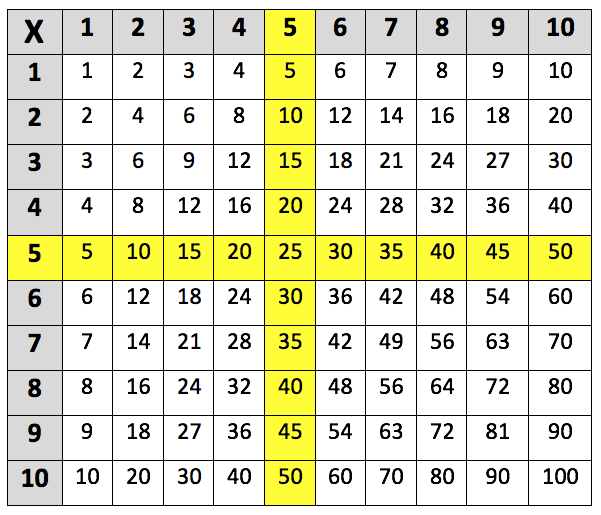Learning the 5 times table. The 5 times table up to 5 x 10 is highlighted in the multiplication grid below:This is the 5 times table up to 5 x 12:
5 x 1 = 5
5 x 2 = 10
5 x 3 = 15
5 x 4 = 20
5 x 5 = 25
5 x 6 = 30
5 x 7 = 35
5 x 8 = 40
5 x 9 = 45
5 x 10 = 50
5 x 11 = 55
5 x 12 = 60

## When do children learn the 5 times table?

In Year 2, children are taught the concept of multiplication and start by learning the 2, 5 and 10 times tables. Children will also learn the division facts for the 5 times table, such as:

5 ÷ 5 = 1 and 10 ÷ 5 = 2

Children are often taught the 2, 5 and 10 times tables first as there are patterns which make them easier to learn. A good knowledge of these times tables can also help children when they go on to learn more difficult times tables.

## How to help children with the 5 times table?

One quick way of identifying multiples of 5 is to know that they always end in either a 5 or a 0. So, it is easy to tell if large numbers are in the 5 times table just by looking at the last digit. For example, the number 5040 is a multiple of 5, as it ends in a 0. Whereas, 4688 is not a multiple of 5 because it ends in an 8.

Another way that children could work out the 5 times table is by multiplying a number by 10 and then halving the answer. This rule works because 5 is half of 10, it would be useful for children who are confident in their 10 times tables. For example, instead of calculating 5 x 7, children could work out that 10 x 7 = 70, and then that half of 70 is 35. Therefore, 5 x 7 = 35.

When you have spare time with your child (such as after dinner), it is good practice to ask them to recite one of their time tables, for example, the 5 times table. This method or recalling can be really helpful, as the repetition will make the times tables stick in a child’s memory. If your child does learn through repetition, you could also invent multiplication songs or rhymes to sing throughout the day.

## How does Learning Street help children with the 5 times table?

Repetition is key to learning your 5 times table, and in the Learning Street 8 year old courses, there is a constant focus on always practising times tables as this is the best way to improve. This is through times tables tests (to improve accuracy), tables races (to improve time), mental mathematics, maths problem solving and other fun activities such as mystery pictures.

Without a good knowledge of times tables, it can lead to problems in SATs, GCSEs and A Levels.

## Our Courses

Click through to review the courses we have available

##### Get Started

Learning Street structured courses make home study easier and more successful. There's no need to wonder which books to buy or what to do next. Everything is planned for you.

• Proven to deliver excellent results
• Used by families, private tutors and schools
• Fully planned and structured